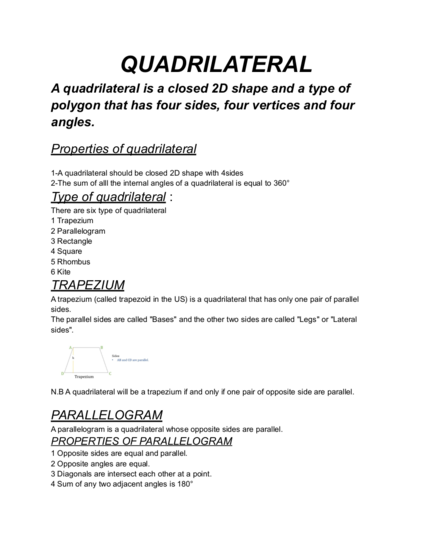Contributed by:A quadrilateral is a closed shape and a type of polygon that has four sides, four vertices, and four angles. It is formed by joining four non-collinear points. The sum of the interior angles of quadrilaterals is always equal to 360 degrees.
A quadrilateral is a closed 2D shape and a type of
polygon that has four sides, four vertices and four
1-A quadrilateral should be closed 2D shape with 4sides
2-The sum of alll the internal angles of a quadrilateral is equal to 360°
There are six type of quadrilateral
1 Trapezium
2 Parallelogram
3 Rectangle
4 Square
5 Rhombus
6 Kite
A trapezium (called trapezoid in the US) is a quadrilateral that has only one pair of parallel
The parallel sides are called "Bases" and the other two sides are called "Legs" or "Lateral
N.B A quadrilateral will be a trapezium if and only if one pair of opposite side are parallel.
A parallelogram is a quadrilateral whose opposite sides are parallel.
PROPERTIES OF PARALLELOGRAM
1 Opposite sides are equal and parallel.
2 Opposite angles are equal.
3 Diagonals are intersect each other at a point.
4 Sum of any two adjacent angles is 180°
2. N.B- A trapezium will be a parallelogram if and only if the legs of trapezium are equal.
A Rectangle is a quadrilateral whose opposite sides are equal and parallel, all the angles are
right angle.
Properties of rectangle
1 Opposite sides are equal and parallel.
2 Every angle is the right angle.
3 Diagonals bisect each other.
4 Diagonals are equal in length.
N.B- (i) A trapezium will be a rectangle if and only if the legs are parallel and every angle are
right angle.
(ii) A parallelogram will be a rectangle if and only if every angle of the parallelogram is the right
Square is a quadrilateral whose every side is equal, opposite sides are parallel and all the
angles are right angle.
Properties of square
1 Every side is equal.
2 Every angle is the right angle.
3 Diagonals are bisect each other perpendicularly.
4 Diagonals are equal.
N.B- (i) Rectangle will be a square if and only if the adjacent sides are equal.
(ii) A rhombus will be a square if and only if at least one angle of the rhombus is right angle.
3. A rhombus is a quadrilateral whose all sides are equal in length and opposite sides are parallel.
N.B- A rhombus with right angles would become a square. Another name for rhombus is the
diamond suit in playing cards.
PROPERTIES OF RHOMBUS
1 Every side is equal.
2 Opposite angles are equal.
3 Opposite sides are parallel.
4 Diagonals bisect each other perpendicularly.
5 Sum of any two adjacent angles is 180°.
A kite is a quadrilateral whose two pair of adjacent sides are equal.
Book a Trial Class
Related Tutorials
Linear and Nonlinear equations worksheet